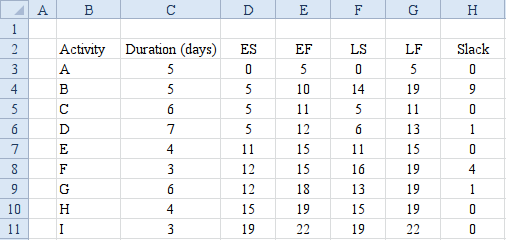## Quiz 4 : Project ManagementLooking for Management Homework Help?# Quiz 4 : Project Management

The dependency matrix of a project is used to represent the dependencies of the activities in a tabular form. The columns represent the information-providing activities and the row rows represent the information-receiving activities. The project network can be represented in terms of a network diagram which is a graphical form of all the project paths. Among several project paths, the longest path(s) are known as the critical path(s). The duration of the critical path(s) is the duration of the overall project. Define the following activity nomenclature for the given project description.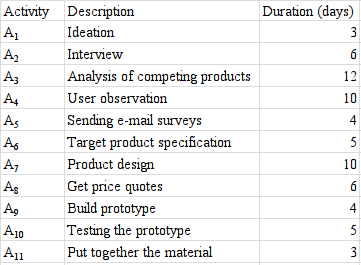a. Considering the dependencies of the activities given, construct the dependency matrix as follows: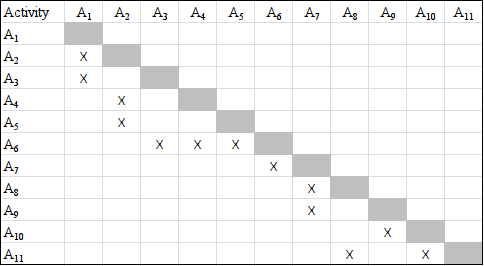b. Use the longest path method to find the critical path as follows: First, draw the network diagram to find the project paths.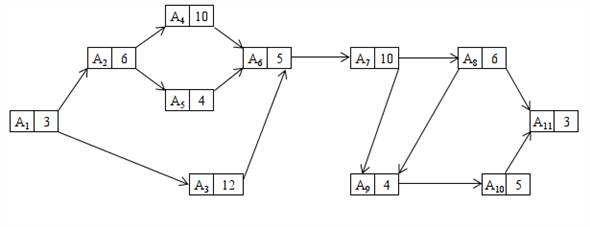Next, recognize the project paths from the network diagram and add the corresponding activity durations to get the path durations.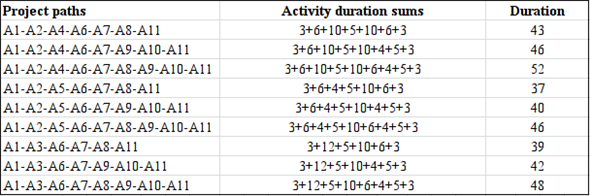Note that the longest path is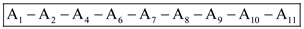. This is the critical path. The duration of the project is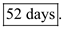The dependency matrix of a project is used to represent the dependencies of the activities in a tabular form. The columns represent the information-providing activities and the row rows represent the information-receiving activities. The project network can be represented in terms of a network diagram which is a graphical form of all the project paths. Among several project paths, the longest path(s) are known as the critical path(s). The duration of the critical path(s) is the duration of the overall project. a. From the given dependency matrix, using the task dependencies, create the project network diagram as follows: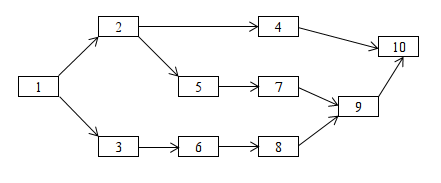b. Find all the project paths from the network and compute the duration of them individually by adding up the durations of the corresponding activities.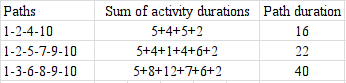Note that the longest path is the third one i.e. the critical path is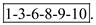The project duration is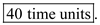c. Compute the Early Start (ES), Early Finish (EF), Late Start (LS), and Late Finish (LF) using the following rules. ES of the starting activities = 0 ES of all other activities = Max. (EF of their immediate predecessors) EF of an activity = Its ES + Its duration LF of ending activities = Max. (All EFs) LF of all other activities = Min. (LS of their immediate successors) LS of an activity = Its LF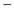Its duration Slack =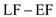Apply the above rules using the following excel formulation to compute the late start, late completion, and slack times.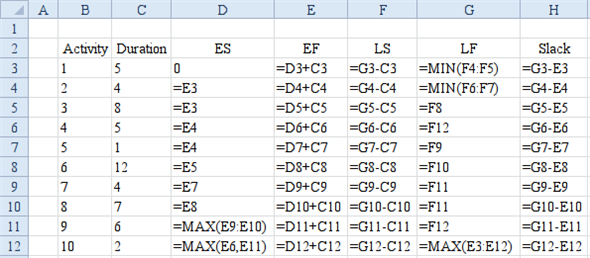Results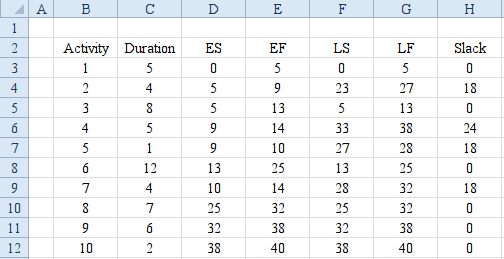The dependency matrix of a project is used to represent the dependencies of the activities in a tabular form. The columns represent the information-providing activities and the row rows represent the information-receiving activities. The project network can be represented in terms of a network diagram which is a graphical form of all the project paths. Among several project paths, the longest path(s) are known as the critical path(s). The duration of the critical path(s) is the duration of the overall project. Define the following activity nomenclature for the given project description.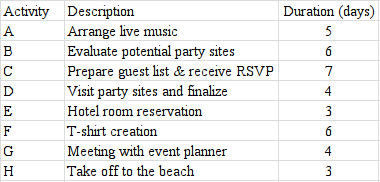a. Considering the dependencies of the activities given, construct the dependency matrix as follows: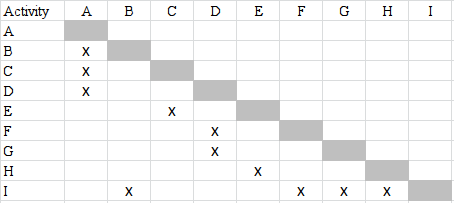b. Use the longest path method to find the critical path as follows: First, draw the network diagram to find the project paths.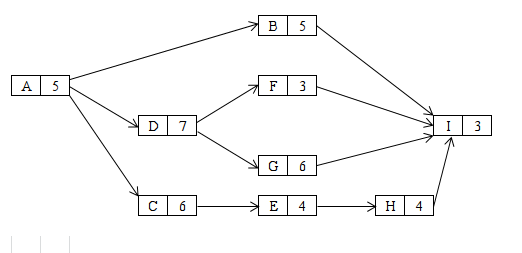c. Recognize the project paths from the network diagram and add the corresponding activity durations to get the path durations.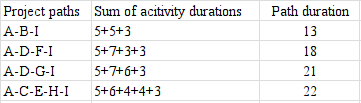Note that the longest path is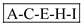. This is the critical path. The duration of the project is 16 days. So, as the final exam starts from 10 th of June, they can go to beach in another 13 days i.e. on 22 nd of June. d. Compute the Early Start (ES), Early Finish (EF), Late Start (LS), and Late Finish (LF) using the following rules. ES of the starting activities = 0 ES of all other activities = Max. (EF of their immediate predecessors) EF of an activity = Its ES + Its duration LF of ending activities = Max. (All EFs) LF of all other activities = Min. (LS of their immediate successors) LS of an activity = Its LFIts duration Slack =Apply the above rules using the following excel formulation to compute the late start, late completion, and slack times.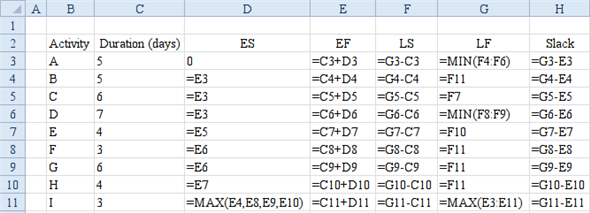Results